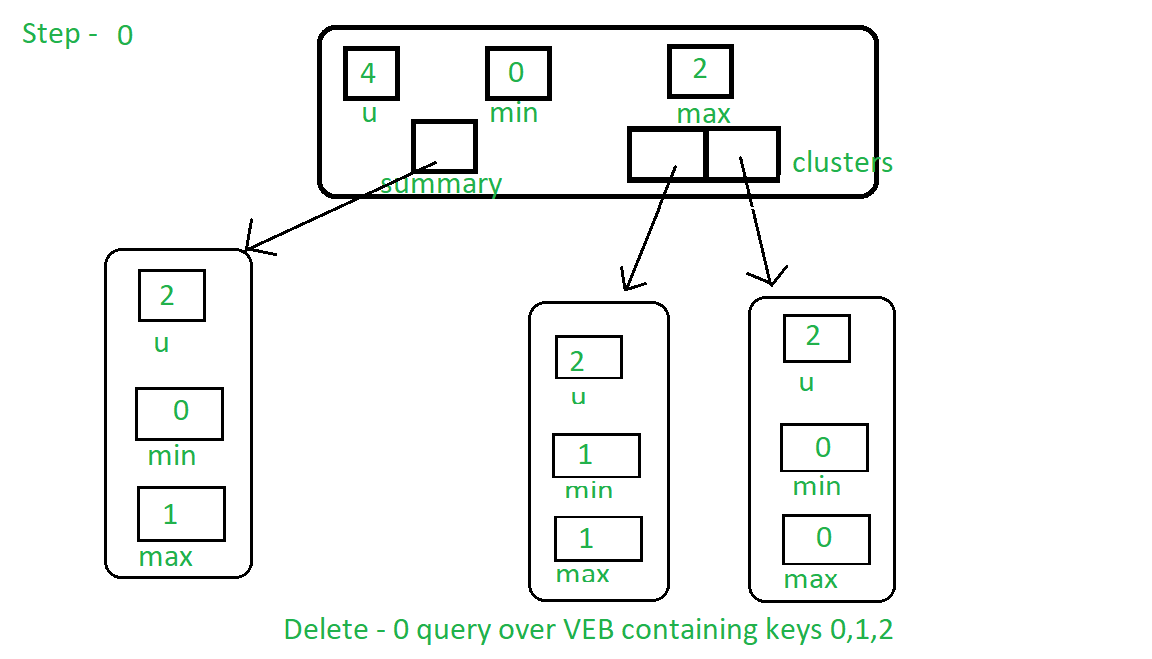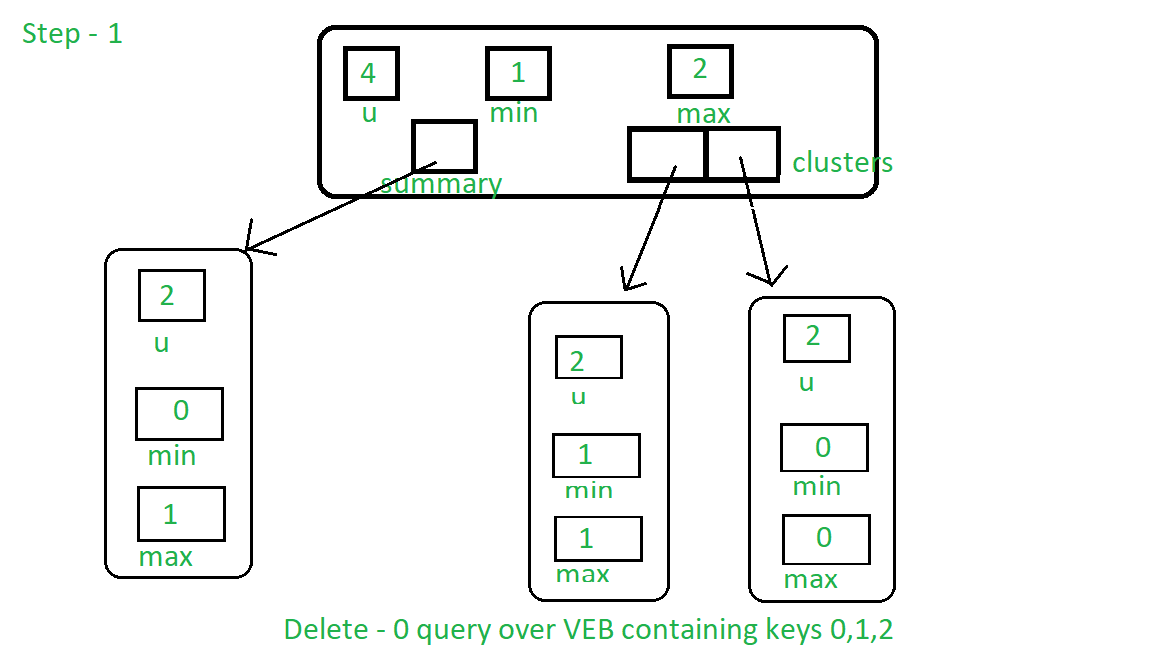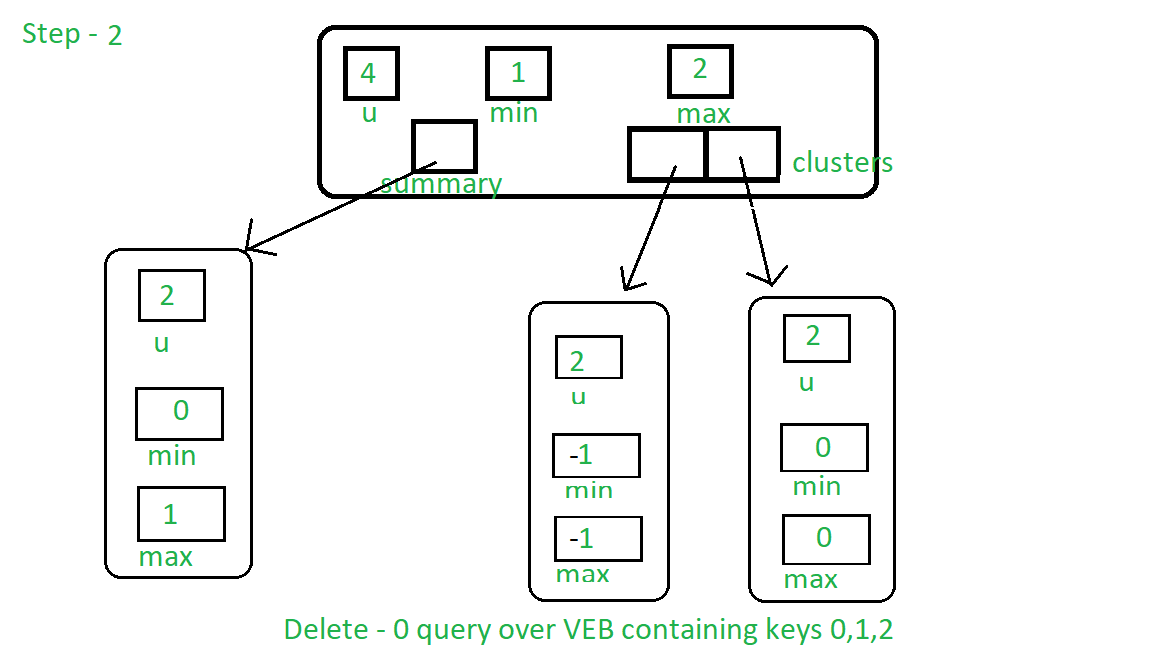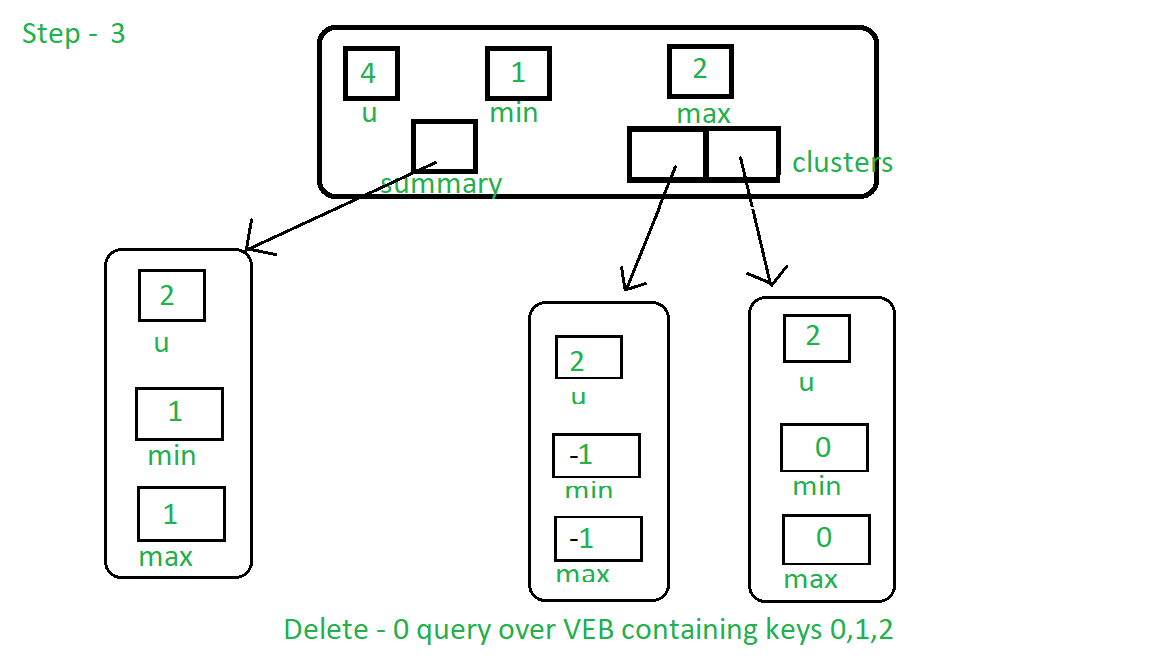# Van Emde Boas Tree | Set 4 | Deletion

It is highly recommended to read the previous articles on Van Emde Boas Tree first.

Procedure for Delete:
Here we are assuming that the key is already present in the tree.

• First we check if only one key is present, then assign the maximum and minimum of the tree to null value to delete the key.
• Base Case: If the universe size of the tree is two then, after the above condition of only one key is present is false, exactly two key is present in the tree (after the above condition turns out to false), So delete the query key by assigning maximum and minimum of the tree to another key present in the tree.
• Recursive Case:
• If the key is the minimum of the tree then find the next minimum of the tree and assign it as the minimum of the tree and delete query key.
• Now the query key is not present in the tree. We will have to change the rest of the structure in the tree to eliminate the key completely:
1. If the minimum of the cluster of the query key is null then we will delete it from summary as well. Also, if the key is the maximum of the tree then we will find new maximum and assign it as the maximum of the tree.
2. Otherwise, if the key is maximum of the tree then find the new maximum and assign it as the maximum of the tree.

Below is the series of images representing ‘delete key-0 query’ over the VEB Tree with 0, 1, 2 keys are present:Step 1: As 0 is the minimum of the tree, it will satisfy the first condition of else part of the algorithm.
First, it finds the next maximum which is 1 and set is as minimum.Step 2: Now it will delete key 1 from the cluster.Step 3: Next condition, cluster has no key, is true, so it will clear the key from the summary as well.`#include ` `using` `namespace` `std; ` ` `  `class` `Van_Emde_Boas { ` ` `  `public``: ` `    ``int` `universe_size; ` `    ``int` `minimum; ` `    ``int` `maximum; ` `    ``Van_Emde_Boas* summary; ` `    ``vector clusters; ` ` `  `    ``// Function to return cluster numbers ` `    ``// in which key is present ` `    ``int` `high(``int` `x) ` `    ``{ ` `        ``int` `div` `= ``ceil``(``sqrt``(universe_size)); ` `        ``return` `x / ``div``; ` `    ``} ` ` `  `    ``// Function to return position of x in cluster ` `    ``int` `low(``int` `x) ` `    ``{ ` `        ``int` `mod = ``ceil``(``sqrt``(universe_size)); ` `        ``return` `x % mod; ` `    ``} ` ` `  `    ``// Function to return the index from ` `    ``// cluster number and position ` `    ``int` `generate_index(``int` `x, ``int` `y) ` `    ``{ ` `        ``int` `ru = ``ceil``(``sqrt``(universe_size)); ` `        ``return` `x * ru + y; ` `    ``} ` ` `  `    ``// Constructor ` `    ``Van_Emde_Boas(``int` `size) ` `    ``{ ` `        ``universe_size = size; ` `        ``minimum = -1; ` `        ``maximum = -1; ` ` `  `        ``// Base case ` `        ``if` `(size <= 2) { ` `            ``summary = nullptr; ` `            ``clusters = vector(0, nullptr); ` `        ``} ` `        ``else` `{ ` `            ``int` `no_clusters = ``ceil``(``sqrt``(size)); ` ` `  `            ``// Assigning VEB(sqrt(u)) to summary ` `            ``summary = ``new` `Van_Emde_Boas(no_clusters); ` ` `  `            ``// Creating array of VEB Tree pointers of size sqrt(u) ` `            ``clusters = vector(no_clusters, nullptr); ` ` `  `            ``// Assigning VEB(sqrt(u)) to all its clusters ` `            ``for` `(``int` `i = 0; i < no_clusters; i++) { ` `                ``clusters[i] = ``new` `Van_Emde_Boas(``ceil``(``sqrt``(size))); ` `            ``} ` `        ``} ` `    ``} ` `}; ` ` `  `// Function to return the minimum value ` `// from the tree if it exists ` `int` `VEB_minimum(Van_Emde_Boas* helper) ` `{ ` `    ``return` `(helper->minimum == -1 ? -1 : helper->minimum); ` `} ` ` `  `// Function to return the maximum value ` `// from the tree if it exists ` `int` `VEB_maximum(Van_Emde_Boas* helper) ` `{ ` `    ``return` `(helper->maximum == -1 ? -1 : helper->maximum); ` `} ` ` `  `// Function to insert a key in the tree ` `void` `insert(Van_Emde_Boas* helper, ``int` `key) ` `{ ` `    ``// If no key is present in the tree ` `    ``// then set both minimum and maximum ` `    ``// to the key (Read the previous article ` `    ``// for more understanding about it) ` `    ``if` `(helper->minimum == -1) { ` `        ``helper->minimum = key; ` `        ``helper->maximum = key; ` `    ``} ` `    ``else` `{ ` `        ``if` `(key < helper->minimum) { ` ` `  `            ``// If the key is less than the current minimum ` `            ``// then swap it with the current minimum ` `            ``// because this minimum is actually ` `            ``// minimum of one of the internal cluster ` `            ``// so as we go deeper into the Van Emde Boas ` `            ``// we need to take that minimum to its real position ` `            ``// This concept is similar to "Lazy Propagation" ` `            ``swap(helper->minimum, key); ` `        ``} ` ` `  `        ``// Not base case then... ` `        ``if` `(helper->universe_size > 2) { ` ` `  `            ``// If no key is present in the cluster then insert key into ` `            ``// both cluster and summary ` `            ``if` `(VEB_minimum(helper->clusters[helper->high(key)]) == -1) { ` `                ``insert(helper->summary, helper->high(key)); ` ` `  `                ``// Sets the minimum and maximum of cluster to the key ` `                ``// as no other keys are present we will stop at this level ` `                ``// we are not going deeper into the structure like ` `                ``// Lazy Propagation ` `                ``helper->clusters[helper->high(key)]->minimum = helper->low(key); ` `                ``helper->clusters[helper->high(key)]->maximum = helper->low(key); ` `            ``} ` `            ``else` `{ ` `                ``// If there are other elements in the tree then recursively ` `                ``// go deeper into the structure to set attributes accordingly ` `                ``insert(helper->clusters[helper->high(key)], helper->low(key)); ` `            ``} ` `        ``} ` ` `  `        ``// Sets the key as maximum it is greater than current maximum ` `        ``if` `(key > helper->maximum) { ` `            ``helper->maximum = key; ` `        ``} ` `    ``} ` `} ` ` `  `// Function that returns true if the ` `// key is present in the tree ` `bool` `isMember(Van_Emde_Boas* helper, ``int` `key) ` `{ ` ` `  `    ``// If universe_size is less than the key ` `    ``// then we can not search the key so returns ` `    ``// false ` `    ``if` `(helper->universe_size < key) { ` `        ``return` `false``; ` `    ``} ` ` `  `    ``// If at any point of our traversal ` `    ``// of the tree if the key is the minimum ` `    ``// or the maximum of the subtree, then ` `    ``// the key is present so returns true ` `    ``if` `(helper->minimum == key || helper->maximum == key) { ` `        ``return` `true``; ` `    ``} ` `    ``else` `{ ` ` `  `        ``// If after attending above condition, ` `        ``// if the size of the tree is 2 then ` `        ``// the present key must be ` `        ``// maximum or minimum of the tree if it ` `        ``// is not then it returns false becuase key ` `        ``// can not be present in the sub tree ` `        ``if` `(helper->universe_size == 2) { ` `            ``return` `false``; ` `        ``} ` `        ``else` `{ ` ` `  `            ``// Recursive call over the cluster ` `            ``// in which the key can be present ` `            ``// and also pass the new position of the key ` `            ``// i.e., low(key) ` `            ``return` `isMember(helper->clusters[helper->high(key)], ` `                            ``helper->low(key)); ` `        ``} ` `    ``} ` `} ` ` `  `// Function to find the successor of the given key ` `int` `VEB_successor(Van_Emde_Boas* helper, ``int` `key) ` `{ ` ` `  `    ``// Base case: If key is 0 and its successor ` `    ``// is present then return 1 else return null ` `    ``if` `(helper->universe_size == 2) { ` ` `  `        ``if` `(key == 0 && helper->maximum == 1) { ` `            ``return` `1; ` `        ``} ` `        ``else` `{ ` `            ``return` `-1; ` `        ``} ` `    ``} ` ` `  `    ``// If key is less then minimum then return minimum ` `    ``// because it will be successor of the key ` `    ``else` `if` `(helper->minimum != -1 && key < helper->minimum) { ` ` `  `        ``return` `helper->minimum; ` `    ``} ` `    ``else` `{ ` ` `  `        ``// Find successor inside the cluster of the key ` `        ``// First find the maximum in the cluster ` `        ``int` `max_incluster = VEB_maximum(helper->clusters[helper->high(key)]); ` ` `  `        ``int` `offset{ 0 }, succ_cluster{ 0 }; ` ` `  `        ``// If there is any key( maximum!=-1 ) present in the cluster then find ` `        ``// the successor inside of the cluster ` `        ``if` `(max_incluster != -1 && helper->low(key) < max_incluster) { ` ` `  `            ``offset = VEB_successor(helper->clusters[helper->high(key)], ` `                                   ``helper->low(key)); ` ` `  `            ``return` `helper->generate_index(helper->high(key), offset); ` `        ``} ` ` `  `        ``// Otherwise look for the next cluster with at least one key present ` `        ``else` `{ ` ` `  `            ``succ_cluster = VEB_successor(helper->summary, helper->high(key)); ` ` `  `            ``// If there is no cluster with any key present ` `            ``// in summary then return null ` `            ``if` `(succ_cluster == -1) { ` `                ``return` `-1; ` `            ``} ` ` `  `            ``// Find minimum in successor cluster which will ` `            ``// be the successor of the key ` `            ``else` `{ ` ` `  `                ``offset = VEB_minimum(helper->clusters[succ_cluster]); ` ` `  `                ``return` `helper->generate_index(succ_cluster, offset); ` `            ``} ` `        ``} ` `    ``} ` `} ` ` `  `// Function to find the predecessor of the given key ` `int` `VEB_predecessor(Van_Emde_Boas* helper, ``int` `key) ` `{ ` ` `  `    ``// Base case: If the key is 1 and it's predecessor ` `    ``// is present then return 0 else return null ` `    ``if` `(helper->universe_size == 2) { ` ` `  `        ``if` `(key == 1 && helper->minimum == 0) { ` `            ``return` `0; ` `        ``} ` `        ``else` `            ``return` `-1; ` `    ``} ` ` `  `    ``// If the key is greater than maximum of the tree then ` `    ``// return key as it will be the predecessor of the key ` `    ``else` `if` `(helper->maximum != -1 && key > helper->maximum) { ` ` `  `        ``return` `helper->maximum; ` `    ``} ` `    ``else` `{ ` ` `  `        ``// Find predecessor in the cluster of the key ` `        ``// First find minimum in the key to check whether any key ` `        ``// is present in the cluster ` `        ``int` `min_incluster = VEB_minimum(helper->clusters[helper->high(key)]); ` ` `  `        ``int` `offset{ 0 }, pred_cluster{ 0 }; ` ` `  `        ``// If any key is present in the cluster then find predecessor in ` `        ``// the cluster ` `        ``if` `(min_incluster != -1 && helper->low(key) > min_incluster) { ` ` `  `            ``offset = VEB_predecessor(helper->clusters[helper->high(key)], ` `                                     ``helper->low(key)); ` ` `  `            ``return` `helper->generate_index(helper->high(key), offset); ` `        ``} ` ` `  `        ``// Otherwise look for predecessor in the summary which ` `        ``// returns the index of predecessor cluster with any key present ` `        ``else` `{ ` ` `  `            ``pred_cluster = VEB_predecessor(helper->summary, helper->high(key)); ` ` `  `            ``// If no predecessor cluster then... ` `            ``if` `(pred_cluster == -1) { ` ` `  `                ``// Special case which is due to lazy propagation ` `                ``if` `(helper->minimum != -1 && key > helper->minimum) { ` `                    ``return` `helper->minimum; ` `                ``} ` ` `  `                ``else` `                    ``return` `-1; ` `            ``} ` ` `  `            ``// Otherwise find maximum in the predecessor cluster ` `            ``else` `{ ` ` `  `                ``offset = VEB_maximum(helper->clusters[pred_cluster]); ` ` `  `                ``return` `helper->generate_index(pred_cluster, offset); ` `            ``} ` `        ``} ` `    ``} ` `} ` ` `  `// Function to delete a key from the tree ` `// assuming that the key is present ` `void` `VEB_delete(Van_Emde_Boas* helper, ``int` `key) ` `{ ` ` `  `    ``// If only one key is present, it means ` `    ``// that it is the key we want to delete ` `    ``// Same condition as key == max && key == min ` `    ``if` `(helper->maximum == helper->minimum) { ` ` `  `        ``helper->minimum = -1; ` `        ``helper->maximum = -1; ` `    ``} ` ` `  `    ``// Base case: If the above condition is not true ` `    ``// i.e. the tree has more than two keys ` `    ``// and if its size is two than a tree has exactly two keys. ` `    ``// We simply delete it by assigning it to another ` `    ``// present key value ` `    ``else` `if` `(helper->universe_size == 2) { ` ` `  `        ``if` `(key == 0) { ` `            ``helper->minimum = 1; ` `        ``} ` `        ``else` `{ ` `            ``helper->minimum = 0; ` `        ``} ` `        ``helper->maximum = helper->minimum; ` `    ``} ` `    ``else` `{ ` ` `  `        ``// As we are doing something similar to lazy propagation ` `        ``// we will basically find next bigger key ` `        ``// and assign it as minimum ` `        ``if` `(key == helper->minimum) { ` ` `  `            ``int` `first_cluster = VEB_minimum(helper->summary); ` ` `  `            ``key ` `                ``= helper->generate_index(first_cluster, ` `                                         ``VEB_minimum(helper->clusters[first_cluster])); ` ` `  `            ``helper->minimum = key; ` `        ``} ` ` `  `        ``// Now we delete the key ` `        ``VEB_delete(helper->clusters[helper->high(key)], ` `                   ``helper->low(key)); ` ` `  `        ``// After deleting the key, rest of the improvements ` ` `  `        ``// If the minimum in the cluster of the key is -1 ` `        ``// then we have to delete it from the summary to ` `        ``// eliminate the key completely ` `        ``if` `(VEB_minimum(helper->clusters[helper->high(key)]) == -1) { ` ` `  `            ``VEB_delete(helper->summary, helper->high(key)); ` ` `  `            ``// After the above condition, if the key ` `            ``// is maximum of the tree then... ` `            ``if` `(key == helper->maximum) { ` `                ``int` `max_insummary = VEB_maximum(helper->summary); ` ` `  `                ``// If the max value of the summary is null ` `                ``// then only one key is present so ` `                ``// assign min. to max. ` `                ``if` `(max_insummary == -1) { ` ` `  `                    ``helper->maximum = helper->minimum; ` `                ``} ` `                ``else` `{ ` ` `  `                    ``// Assign global maximum of the tree, after deleting ` `                    ``// our query-key ` `                    ``helper->maximum ` `                        ``= helper->generate_index(max_insummary, ` `                                                 ``VEB_maximum(helper->clusters[max_insummary])); ` `                ``} ` `            ``} ` `        ``} ` ` `  `        ``// Simply find the new maximum key and ` `        ``// set the maximum of the tree ` `        ``// to the new maximum ` `        ``else` `if` `(key == helper->maximum) { ` ` `  `            ``helper->maximum ` `                ``= helper->generate_index(helper->high(key), ` `                                         ``VEB_maximum(helper->clusters[helper->high(key)])); ` `        ``} ` `    ``} ` `} ` ` `  `// Driver code ` `int` `main() ` `{ ` `    ``Van_Emde_Boas* end = ``new` `Van_Emde_Boas(8); ` ` `  `    ``// Inserting Keys ` `    ``insert(end, 1); ` `    ``insert(end, 0); ` `    ``insert(end, 2); ` `    ``insert(end, 4); ` ` `  `    ``// Before deletion ` `    ``cout << isMember(end, 2) << endl; ` `    ``cout << VEB_predecessor(end, 4) << ``" "` `         ``<< VEB_successor(end, 1) << endl; ` ` `  `    ``// Delete only if the key is present ` `    ``if` `(isMember(end, 2)) ` `        ``VEB_delete(end, 2); ` ` `  `    ``// After deletion ` `    ``cout << isMember(end, 2) << endl; ` `    ``cout << VEB_predecessor(end, 4) << ``" "` `         ``<< VEB_successor(end, 1) << endl; ` `} `

Output:

```1
2 2
0
1 4
```

GeeksforGeeks has prepared a complete interview preparation course with premium videos, theory, practice problems, TA support and many more features. Please refer Placement 100 for details

My Personal Notes arrow_drop_upCheck out this Author's contributed articles.

If you like GeeksforGeeks and would like to contribute, you can also write an article using contribute.geeksforgeeks.org or mail your article to contribute@geeksforgeeks.org. See your article appearing on the GeeksforGeeks main page and help other Geeks.

Please Improve this article if you find anything incorrect by clicking on the "Improve Article" button below.